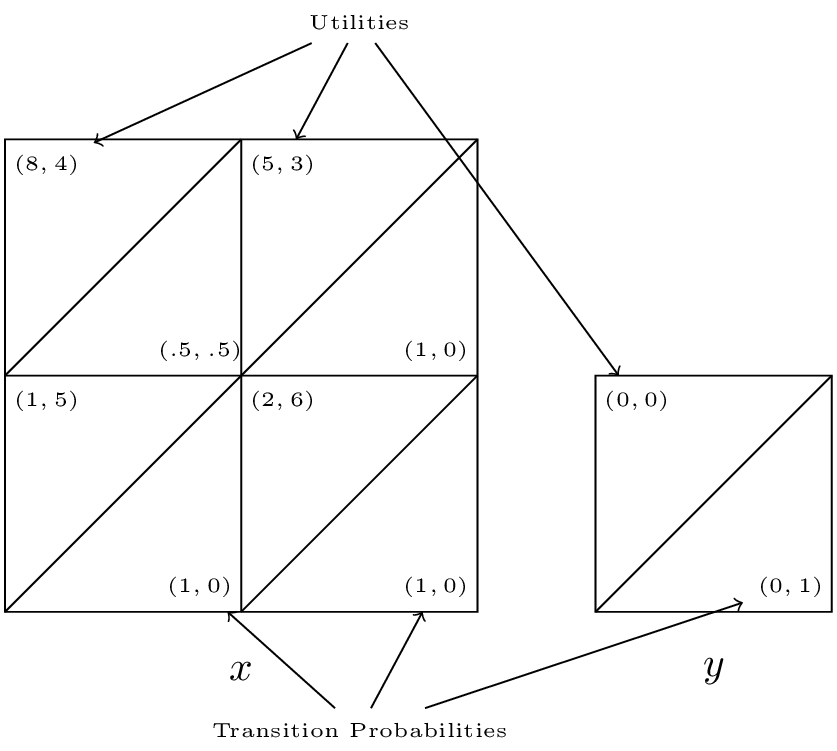## Recap

In the previous chapter:

• We considered games of incomplete information;
• Discussed some basic utility theory;
• Considered the principal agent game.

In this chapter we will take a look at a more general type of random game.

## Stochastic games

### Definition of a stochastic game

A stochastic game is defined by:

• X a set of states with a stage game defined for each state;
• A set of strategies $$S_i(x)$$ for each player for each state $$x\in X$$;
• A set of rewards dependant on the state and the actions of the other players: $$u_i(x,s_1,s_2)$$;
• A set of probabilities of transitioning to a future state: $$\pi(x’|x,s_1,s_2)$$;
• Each stage game is played at a set of discrete times $$t$$.

We will make some simplifying assumptions in this course:

1. The length of the game is not known (infinite horizon) - so we use discounting;
2. The rewards and transition probabilities are not dependent;
3. We will only consider strategies called Markov strategies.

### Definition of a Markov strategy

A strategy is call a Markov strategy if the behaviour dictated is not time dependent.

### Example

Consider the following game with $$X=\{x,y\}$$:

• $$S_1(x)=\{a,b\}$$ and $$S_2(x)=\{c,d\}$$;
• $$S_1(y)=\{e\}$$ and $$S_2(x)=\{f\}$$;

We have the stage game corresponding to state $$x$$:

The stage game corresponding to state $$y$$:

The transition probabilities corresponding to state $$x$$:

The transition probabilities corresponding to state $$y$$:

A concise way of representing all this is shown.We see that the Nash equilibrium for the stage game corresponding to $$x$$ is $$(a, c)$$ however as soon as the players play that strategy profile they will potentially go to state $$y$$ which is an absorbing state at which players gain no further utility.

To calculate utilities for players in infinite horizon stochastic games we use a discount rate. Thus without loss of generality if the game is in state $$x$$ and we assume that both players are playing $$\sigma^*_i$$ then player 1 would be attempting to maximise future payoffs:

where $$U_1^*$$ denotes the expected utility to player 1 when both players are playing the Nash strategy profile.

Thus a Nash equilibrium satisfies:

Solving these equations is not straightforward. We will take a look at one approach by solving the example we have above.

## Finding equilibria in stochastic games

Let us find a Nash equilibrium for the game considered above with $$\delta=2/3$$.

State $$y$$ gives no value to either player so we only need to consider state $$x$$. Let the future gains to player 1 in state $$x$$ be $$v$$, and the future gains to player 2 in state $$x$$ be $$u$$. Thus the players are facing the following game:

We consider each strategy pair and state the condition for Nash equilibrium:

1. $$(a,c)$$: $$v\leq 21$$ and $$u\leq 3$$.
2. $$(a,d)$$: $$u\geq3$$.
3. $$(b,c)$$: $$v\geq 21$$ and $$5\geq 6$$.
4. $$(b,d)$$: $$5\leq2$$.

Now consider the implications of each of those profiles being an equilibrium:

1. $$8+v/3=v$$ $$\Rightarrow$$ $$v=12$$ and $$4+u/3=u$$ $$\Rightarrow$$ $$u=6$$ which contradicts the corresponding inequality.
2. $$3+2u/3=u$$ $$\Rightarrow$$ $$u=9$$.
3. The second inequality cannot hold.
4. The inequality cannot hold.

Thus the unique Markov strategy Nash equilibria is $$(a,d)$$ which is not the stage Nash equilibria!